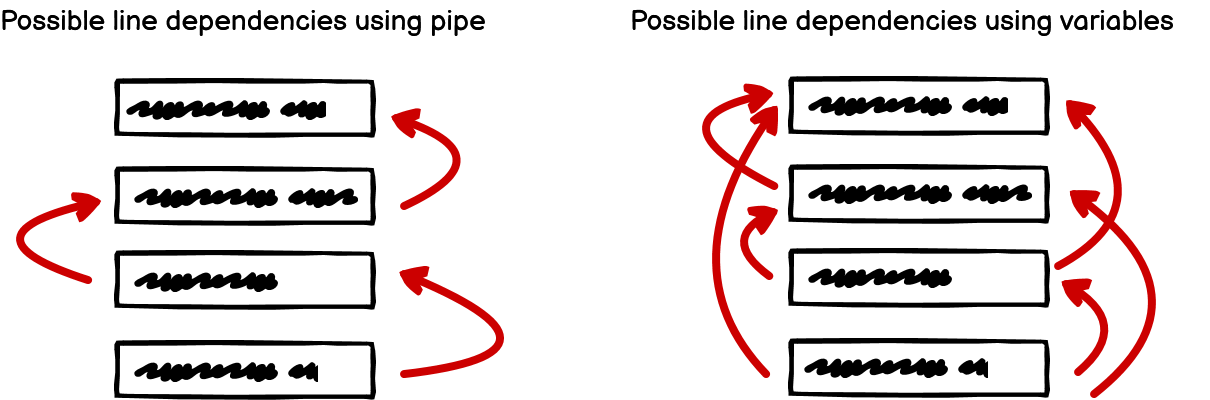Seite wählen

# My favorite TypeScript function

von | 26. April 2023 | English, Software Engineering, Tools & Frameworks### Moritz Rumpf

Writing clean code is an important part of modern software development. In this article, our colleague Moritz presents his favorite function “pipe” which helps him write clean code in Typescript projects.

Over the past years, I have worked on a few TypeScript codebases, each different in its own way. One of the things they all had in common, though, was that they all profited from the addition of one simple function.

In this article I will tell you about my favorite TypeScript function `pipe`. It’s easy to introduce and useful in many situations.

Let’s start with a small coding task to see where `pipe` can help us.

From an array of dice rolls (for example `[1,6,3,6,3,1,3,2]`) return the sum of all even rolls where the digits are interspersed with ‘-‘. The result of the example should be `1-4`.

Some functions are already present in the code base:

• `sum` calculates the sum from an array of numbers
• `isEven` returns true if the input number is even, false otherwise
• `intersperseWithDash` takes in a string and intersperses it with dashes

A solution to the task could be the following program:

``````const result = intersperseWithDash(
sum(
rolls
.filter(isEven)
).toString()
)``````

It uses our predefined functions, so it is relatively concise.

One issue with the above code is the awkward order in which you have to read the lines. If you want to read the lines in the order that they are applied to the input, you have to read them as follows:

``````const result = intersperseWithDash( // 5.
sum( // 3.
rolls // 1.
.filter(isEven) // 2.
).toString() // 4.
)``````

We start somewhere in the middle and then jump to the top, then to the bottom, and then to the top again.

What if we could read it from top to bottom? We can do that with methods like `map`, `filter`, or `toString` because these are present on the object we are operating on, but `sum` and `intersperseWithDash` are not.

## Introducing `pipe`

Let’s see how the order of lines changes when we introduce `pipe`.

``````const result = pipe(
rolls.filter(isEven),
sum,
theSum => theSum.toString(),
intersperseWithDash
)``````

If we define our own `filter` and `toString` functions, we can make this even more concise.

``````const result = pipe(
rolls,
filter(isEven),
sum,
toString,
intersperseWithDash
)``````

We now have an easier time visualizing what is happening in each step:

``````const result = pipe(
rolls, // [1,6,3,6,3,1,3,2]
filter(isEven), // [6,6,2]
sum, // 14
toString, // '14'
intersperseWithDash // '1-4'
)``````

Now, you might be thinking “wait, I can also extract each step into a variable”, and rightfully so!

## An alternative way

Let’s see how the code looks when we extract each line into a variable.

``````const evenRolls = rolls.filter(isEven)
const sumOfEvenRolls = sum(evenRolls)
const sumAsString = sumOfEvenRolls.toString()
const result = intersperseWithDash(sumAsString)``````

The result of each step is now named, allowing us to describe each intermediate result in more detail, great!

There is a catch, though. Nothing is enforcing the top-to-bottom flow. Every line can now depend on each line above it, making it harder to quickly understand the flow of data at a glance.With `pipe` I can mostly forget about everything but the current line I am reading and the line before it.

## How `pipe` works

For simplicity’s sake, we can look at the definition with 2 functions (instead of any number of functions).

``const pipe = a => ab => bc => bc(ab(a))``

It takes in an initial argument and some unary functions which it executes one after the other, that’s it!

The complete definition of `pipe` contains a bit of boilerplate. Libraries like fp-ts or ts-belt provide a type definition for `pipe` which you can copy or include.

## Conclusion

We saw how `pipe` can enforce a top-to-bottom control flow, making it easier to follow the flow of the data.

If that got you excited, I encourage you to try this out yourself.

Or you can take `pipe` as the starting step to dive into the whole world of functional programming and discover universally useful concepts such as the do-notation.